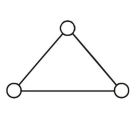# Prove the following

Question:Consider a gas of triatomic molecules. The molecules are assumed to the triangular and made of massless rigid rods whose vertices are occupied by atoms. The internal energy of a mole of the gas at temperature $\mathrm{T}$ is :

1. $\frac{9}{2} \mathrm{RT}$

2. $\frac{3}{2} \mathrm{RT}$

3. $\frac{5}{2} \mathrm{RT}$

4. $3 \mathrm{RT}$

Correct Option: , 4

Solution:

$\mathrm{DOF}=3+3=6$

$\mathrm{U}=\frac{\mathrm{f}}{2} \mathrm{nRT}=3 \mathrm{RT}$